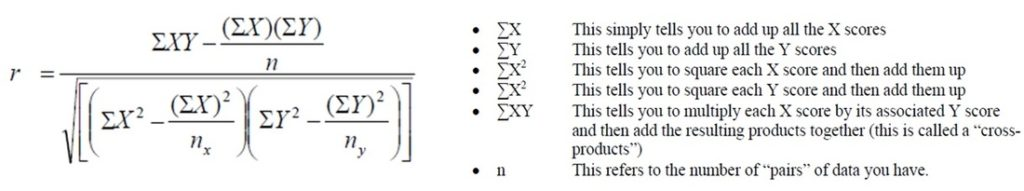# Karl Pearson’s Algorithm in Python

Karl Pearson’s Correlation:

To deduce the degree of correlation between two variables, Karl Pearson deduced a formula in 1896.

To break this up, it means ” Pearson’s Correlation co-efficient” is :-

•  useful to measure how strong a relationship is between two variables,
•  commonly used in linear regression.

..basically it concerns with collection, manipulation, management, organization and analysis of numeric data.

What is it used for ?

Much used for data fitting in all sciences, to tell how related two or more variables under consideration are:-Ok, alright !! When you,

1. Want to see relation between your diet and weight? ….Use Karl Pearson’s correlation
This is a positive relationship as variables change in same direction. So is, the relationship between your height and weight (yes !!…I just judged you.) and also between your diet and weight
2. Want to see relation between Price and quantity in demand ? ….Use Karl Pearson’s correlation
This is a negative relationship as variables change in opposite direction.  So is, alcohol consumption and driving ability.

But how exactly do you find a relationship ?

Without the alarm, you probably would have overslept. In this scenario, the alarm had the effect of you waking up at a certain time. This is what is meant by cause and effect. A causeeffect relationship helps you to find the relationship between two variables. This causeeffect relationship is a relationship in which one event (the cause) makes another event happen (the effect). Also, called as Causation

Assumptions:-

1. Linear Relationship — the relationship between the variables must be ‘linear’ that is when the data is plotted, it tends to cluster around a non horizontal straight line and if it does not, it can be nonlinear.
2. Normal distribution — There has to be a large number of independent causes that affect the variables under study so as to form a Normal Distribution.
The normal distribution can be characterized by the mean and standard deviation. The mean determines where the peak occurs. The standard deviation is a measure of the spread of the normal probability distribution.

Linear Correlation can be of Three types:-

Correlation co-efficient value always lie between ±1. Types are as follows:

1. Positive Correlation: (+1) when values of one variable increase with that of another. In simple words, the date makes a straight line going through the origin (0,0) to the increasing values of X and Y.
2. Negative Correlation: (-1) when increase in value of one variable causes corresponding values of another variable to decrease. In simple words, data makes a straight line going through the higher values of Y down to the higher values of X.
3. No Correlation: No impact on one variable with increase/decrease of values of another variable for example: your age(X) and Internet Bandwidth(Y).

Using Python for calculating Correlation between AGE and GLUCOSE LEVEL :-

Consider two variables i.e. Age(X) and Glucose Level (Y), the correlation between these variables refers to their relationship between them in a way that in which manner they will vary.

 S.No. Age (X) Glucose Level (Y) 1 47 99 2 27 55 3 38 63 4 34 59 5 45 87 6 59 81

The formula that helps us deduce the correlation is:-Our output comes out to be 0.742 , proving that the variables have a moderate positive correlation.